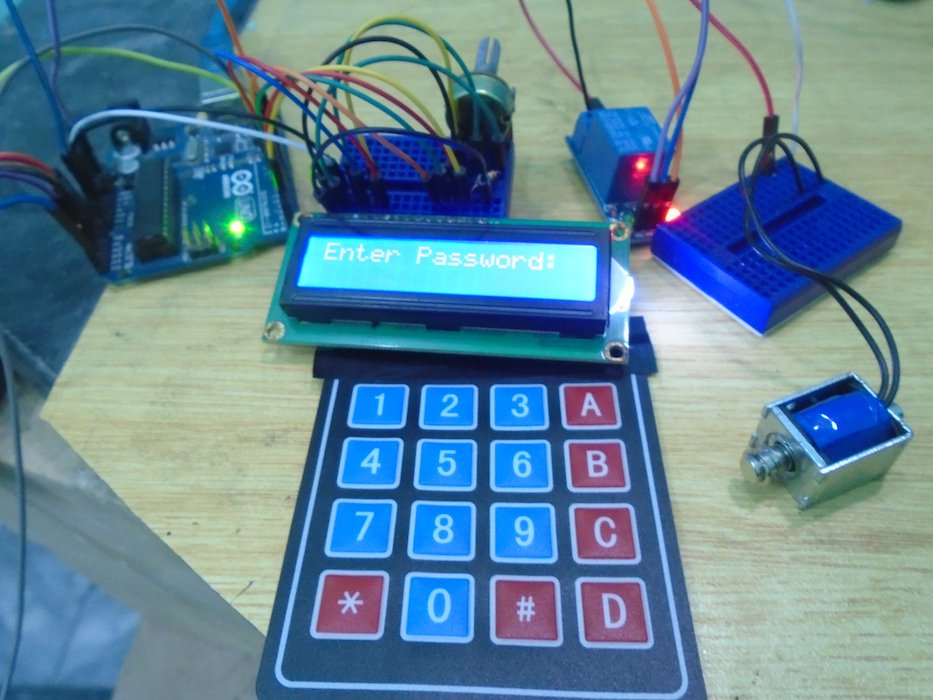Use an Arduino to make your own keyless door lock system with LED display.

In this project, we are going to make a keyless door lock system which uses a 4X4 keypad to enter the keys and a DC lock to open or close the door. A 16X2 LCD will be used for display.

### How Does the Keyless Door Lock System Work?

Whenever the keys are pressed, they are matched with the keys already stored. If the keys that are pressed match the initial password stored in the EEPROM which is ‘1234’, then the lock will open up. If the password does not match, then it will print “access denied” on the LCD.

If the ‘#’ key will be pressed, it will ask you to enter the current password and if it matches, then it will ask you to enter the new password and the password will be changed.### Circuit Diagram

First, connect the 4X4 keypad to the Arduino; connect the first six pins on the 4X4 keypad with the A0 and A5 pins on the Arduino. Then connect the last two pins on the 4X4 keypad module to digital pins 3 and 2 on the Arduino.

After that, connect the LCD to the Arduino. The connections for connecting the LCD with the Arduino are as follows:

• Connect pin 1 on the LCD, which is the VSS pin, to GND on the Arduino
• Connect pin 2, which is the VDD pin, to the 5V pin on the Arduino
• Connect pin 3, which is the V0, to the middle of the 10k potentiometer and connect the other two pins on the potentiometer to 5V and GND on the Arduino. This pin is for setting the LCD's contrast.
• Connect pin 4, which is the RS pin, to pin 7 on the Arduino
• Connect pin 5, which is the R/W pin, to the GND pin on the Arduino
• Connect pin 6, which is the Enable pin, to pin 6 on the Arduino
• Connect pins 11, 12, 13, and 14 which are the data pins, to the pins 5, 4, 3, and 2 on the Arduino
• Connect pin 15, which is the LCD's backlight pin, to 5V on the Arduino through the 220-ohm resistor
• Connect pin 16 on the Arduino, which is the negative pin of the backlight, to GND on the Arduino

Last, we will connect the DC lock with the Arduino. The Lock operates on a voltage from 7 to 12V, so we cannot directly connect it to the Arduino. To connect it to the Arduino, we will require a relay and a battery.

Connect the signal pin of the relay to pin 10 on the Arduino and the lock's VCC and GND to 5V and GND on the Arduino. Then on the other end of the relay, connect the negative of the battery to the common on the relay and the NO (Normally open) on the relay to one side of the lock. Then connect the other side of the lock to the positive terminal on the battery.```#include <Keypad.h>

#include<LiquidCrystal.h>

#include<EEPROM.h>

LiquidCrystal liquid_crystal_display(9,8,7,6,5,4,);

int i=0;

int relay_pin = 10;

char key_pressed=0;

const byte rows = 4;

const byte columns = 4;

char hexaKeys[rows][columns] = {

{'1','2','3','A'},

{'4','5','6','B'},

{'7','8','9','C'},

{'*','0','#','D'}

};

byte row_pins[rows] = {A0,A1,A2,A3};

byte column_pins[columns] = {A4,A5,3,2};

void setup()

{

pinMode(relay_pin, OUTPUT);

liquid_crystal_display.begin(16,2);

liquid_crystal_display.print(" DIYhacking.com");

liquid_crystal_display.setCursor(0,1);

liquid_crystal_display.print("Electronic Lock ");

delay(2000);

liquid_crystal_display.clear();

liquid_crystal_display.setCursor(0,1);

}

void loop()

{

digitalWrite(relay_pin, HIGH);

if(key_pressed=='#')

change();

if (key_pressed)

{

liquid_crystal_display.print(key_pressed);

}

if(i==4)

{

delay(200);

for(int j=0;j<4;j++)

{

liquid_crystal_display.clear();

liquid_crystal_display.print("Pass Accepted");

digitalWrite(relay_pin, LOW);

delay(2000);

liquid_crystal_display.setCursor(0,1);

liquid_crystal_display.print("Pres # to change");

delay(2000);

liquid_crystal_display.clear();

liquid_crystal_display.setCursor(0,1);

i=0;

}

else

{

digitalWrite(relay_pin, HIGH);

liquid_crystal_display.clear();

liquid_crystal_display.setCursor(0,1);

liquid_crystal_display.print("Pres # to Change");

delay(2000);

liquid_crystal_display.clear();

liquid_crystal_display.setCursor(0,1);

i=0;

}

}

}

void change()

{

int j=0;

liquid_crystal_display.clear();

liquid_crystal_display.setCursor(0,1);

while(j<4)

{

if(key)

{

liquid_crystal_display.print(key);

}

key=0;

}

delay(500);

{

liquid_crystal_display.clear();

liquid_crystal_display.setCursor(0,1);

liquid_crystal_display.print("Try Again");

delay(1000);

}

else

{

j=0;

liquid_crystal_display.clear();

liquid_crystal_display.setCursor(0,1);

while(j<4)

{

if(key)

{

liquid_crystal_display.print(key);

EEPROM.write(j,key);

j++;

}

}

liquid_crystal_display.print("Pass Changed");

delay(1000);

}

liquid_crystal_display.clear();

liquid_crystal_display.setCursor(0,1);

key_pressed=0;

}

for(int j=0;j<4;j++)

EEPROM.write(j, j+49);

for(int j=0;j<4;j++)

}

```

### Code Explanation

First of all, we will include the libraries for the 4X4 keypad, LCD, and a library for storing the password. The EEPROM library is used to store the password. The default password stored in it is ‘1234’.

```#include <Keypad.h>

#include<LiquidCrystal.h>

#include<EEPROM.h>

```

In the loop function, the key pressed will be stored in the ‘key_pressed’ variable and it will be compared with the other keys. If the pressed key is ‘#’, then the change function will be called and it will ask for the new password.

```key_pressed = keypad_key.getKey();

if(key_pressed=='#')

change();```

The keys pressed are stored in the ‘password’ variable and these are shown on the LCD when the keys are pressed. Then these keys will be matched with the initial password stored in the EEPROM. If the keys that were pressed match the initial password, then the lock will get open and “Pass accepted” will be printed on the LCD. If the password does not match, then it will ask to enter the password again.

```if (key_pressed)

{

liquid_crystal_display.print(key_pressed);

}

if(i==4)

{

delay(200);

for(int j=0;j<4;j++)

{

liquid_crystal_display.clear();

liquid_crystal_display.print("Pass Accepted");

digitalWrite(relay_pin, LOW);

delay(2000);
```

If the ‘#’ key is pressed, then it will call the ‘change()’ function. In the change function, it will ask you to enter the current password. If the current password is correct, then it will ask you to enter the new password. Upon entering the new password, the password will be changed.

```void change()

{

int j=0;

liquid_crystal_display.clear();

liquid_crystal_display.setCursor(0,1);

while(j<4)

{

if(key)

{

liquid_crystal_display.print(key);

}

key=0;

}

delay(500);```

Interfacing 16X2 lcd with Arduino

Interfacing 16X2 lcd with Arduino